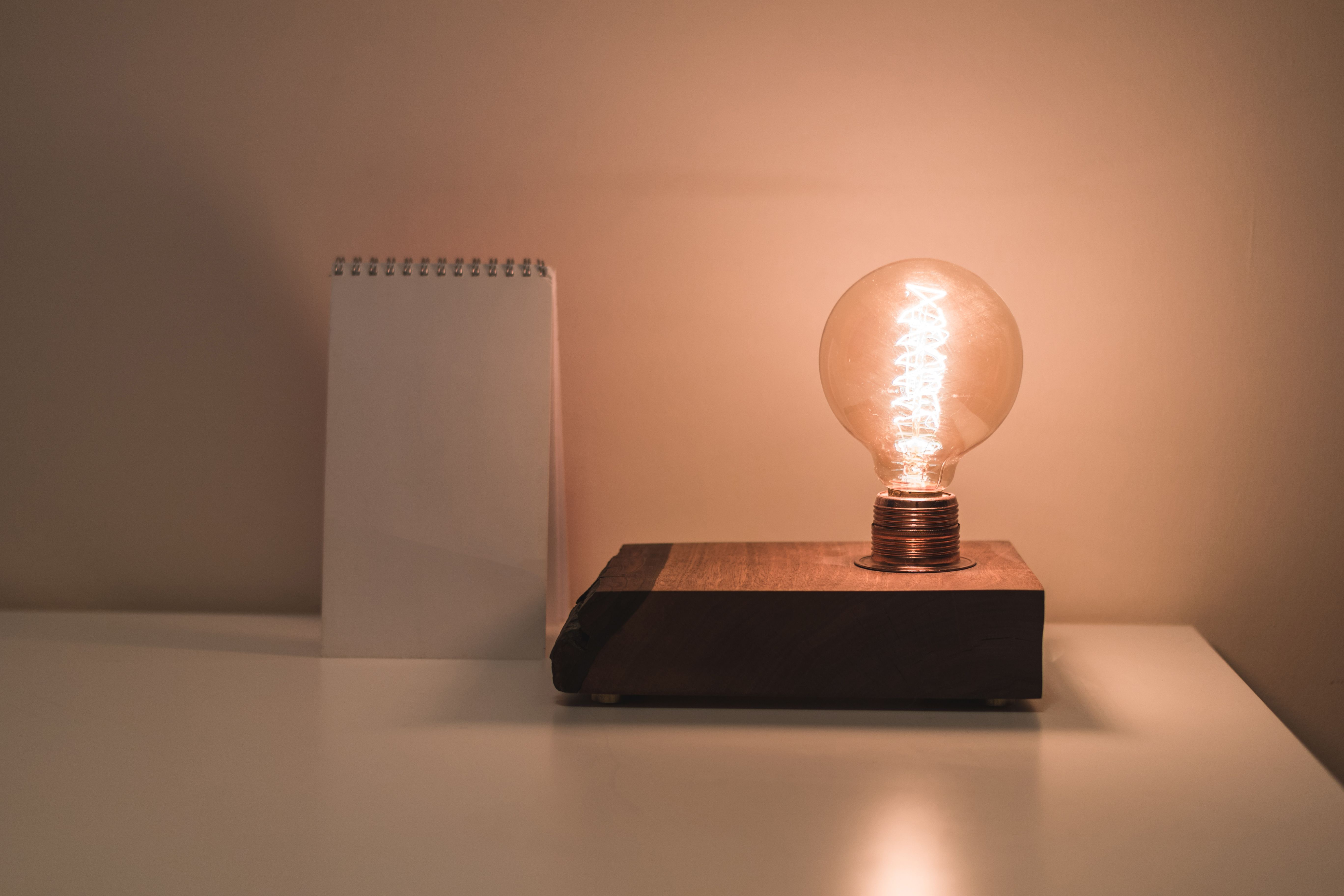# What is ohm's lawOhm's Law is a fundamental principle in electrical engineering and physics that describes the relationship between voltage, current, and resistance in an electrical circuit. It states that the current flowing through a conductor between two points is directly proportional to the voltage across the two points and inversely proportional to the resistance of the conductor.

Mathematically, Ohm's Law is represented as:

V=I×R

where:

• $V$ represents voltage (in volts)
• $I$ represents current (in amperes)
• $R$ represents resistance (in ohms)

In simpler terms, Ohm's Law tells us that the current flowing through a conductor is determined by the voltage applied and the resistance of the conductor.

Diagram: Imagine a simple circuit consisting of a resistor (R) connected to a voltage source (V):

   + ----[ R ]---- -
|              |
|              |
Voltage        Current
Source          Flow

• The "+" terminal of the voltage source is connected to one end of the resistor, and the "-" terminal is connected to the other end.
• The flow of current (I) passes through the resistor from the "+" terminal to the "-" terminal.
• The voltage across the resistor is denoted as V.

According to Ohm's Law, $V=I×R$:

• The voltage across the resistor (V) is equal to the current (I) flowing through it multiplied by the resistance (R) of the resistor.

For example, if you have a resistor with a resistance of 10 ohms and a voltage of 20 volts is applied across it: $V=20\text{\hspace{0.17em}}\text{V}$, $R=10\text{\hspace{0.17em}}\mathrm{\Omega }$

Using Ohm's Law:

So, the current flowing through the resistor is 2 amperes.

Ohm's Law provides a fundamental basis for understanding and analyzing electrical circuits, helping engineers and scientists predict the behavior of components and systems.

Prasun Barua

Prasun Barua is an Engineer (Electrical & Electronic) and Member of the European Energy Centre (EEC). His first published book Green Planet is all about green technologies and science. His other published books are Solar PV System Design and Technology, Electricity from Renewable Energy, Tech Know Solar PV System, C Coding Practice, AI and Robotics Overview, Robotics and Artificial Intelligence, Know How Solar PV System, Know The Product, Solar PV Technology Overview, Home Appliances Overview, Tech Know Solar PV System, C Programming Practice, etc. These books are available at Google Books, Google Play, Amazon and other platforms.

*